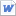검색어 입력폼

acid-base titration

저작시기 2014.03 |등록일 2017.03.25MS 워드 (docx) | 2페이지 | 가격 2,000원

목차

1. Introduction
2. Experimental
3. Results & Discussion
4. Conclusion
5. Reference

본문내용

1. Introduction
Molarity (M) or molar concentration is a common unit for expressing the concentration of solutions. It is defined as the number of moles of solute per liter of solution.

Molarity (M) = moles solute / volume (liter) solution

The concentration of a basic solution can be determined by titrating it with a volume of a standard acid solution required to neutralize it.

The purpose of the titration is the detection of the equivalence point, the point at which chemically equivalent amounts of the reactants have been mixed.

참고 자료

http://www.chem.wisc.edu/deptfiles/genchem/sstutorial/Text11/Tx113/tx113.html